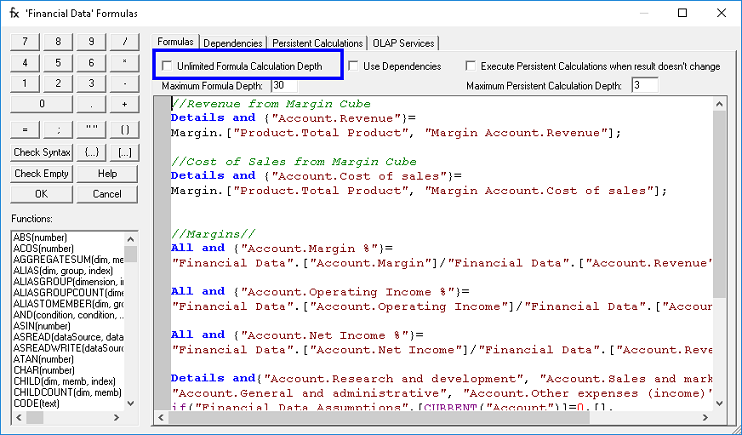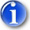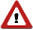Calculation DepthUnlimited Calculation Depth

This feature allows PowerOLAP® to calculate “deep” formulas by checking the option “Unlimited Calculation Depth” in the Formula Editor interface, as shown below.In previous versions of PowerOLAP®, a recursive or circular formula would produce an error displayed as #DEPTH in the cells of a Slice if the formula was not populated with values after 30 levels of recursion. A recursive formula means that the formula refers back to its own internal elements, when a Member on the left hand side of the formula is set to equal itself, either directly or indirectly defined, on the right hand side of the formula.

An example of a recursive formula is one for daily opening balance where today’s opening balance equals yesterday’s closing balance. This model would require a Day dimension and the measures opening balance and closing balance. The formula would state:

 Current day opening balance = (Current day – 1) Closing balance

Current day – 1 is the same as yesterday. The formula dictates that yesterday’s opening balance equals the day before’s closing balance, and so on, until we reach day 1 in the Day dimension.  In order to return a result, the formula would have to cycle through all of the previous days before it reached a value with which it could populate the current day. However, after calculating this balance for 30 days you would reach 30 levels of recursion, which would normally return a #DEPTH error unless Unlimited Calculation Depth is enabled. When enabled, Unlimited Calculation Depth will return the correct data to the current day opening balance cells no matter how many days there are in the model.ImportantThe Unlimited Calculation Depth feature is for a formula where you know the calculation will finish at some point, but after more than 30 levels of recursion. When Unlimited Calculation Depth is enabled, these formulas would continue beyond the 30-level recursion barrier and finish at the first value to return the correct answer in the cells.WarningIf there is no “bottom” to a calculation, PowerOLAP® will hang. A good example of a recursive formula situation that would NOT work with Unlimited Calculation Depth is the following pair of formulas: one for Revenue, where Revenue = Units x Price, and the second for Price where Price = Revenue / Price. In the second formula, Price is defined by Revenue, which in turn was defined by Price in the first formula. This formula would never finish calculating and it is a true recursive loop without an end. If the Unlimited Calculation Depth feature were turned on, the formulas would never finish calculating.

Maximum Calculation Depth for Formulas and Persistent Calculations

Maximum Calculation Depth allows a user to specify how many times formulas can re-enter the calculation engine before an error is returned.  Re-calculation occurs when the calculation of a LHS (left hand side) requires the value needed on the RHS (right hand side) to be calculated from a formula.  For example, if there are two formulas:

 A = b*c     C = d*e

A is calculated by multiplying b*c.  However, c is a formula calculated value.  In order for the engine to calculate A, it must calculate C.  This calculation requires a depth of 2.  If d or e was a formula calculated value then the depth would become 3.  By default, the calculation engine allows 30 levels of depth before returning an error.  The reason for this feature is that a user can develop a non-terminating recursive function.  For example:

 A = b*c     C = d*e     D = a*g

In the above example, A depends on C, then C depends on D, then D depends on A….This will create an infinite loop and if there is no depth limit, the application will crash.

There may, however, be cases when you will want significant depth for formulas.  For example, you may be calculating YTD values across multiple years.  Doing this could create a formula calculation that easily exceeds the 30 limit but still ends properly.

Maximum Persistent Calculation Depth allows a user to limit the number of times a persistent calculation loops.  Looping persistent calculations is a very powerful feature of persistent calculations.  For example, you may want your model to change A if the user changes B, or change B if the user changes A.  Normally this type of formula would recalculate infinitely until the application crashes.  The maximum persistent calculation depth allows a user to define how many times to recalculate through persistent calculations until stopping.  In the above example the maximum level necessary to work correctly is one.  That means that if A changes, it should change B then stop.  There is no need to then change A since that is the value the user changed.  If your model goes A->B->C then the depth is 2 meaning that if A changes, B must change, and if B changes, then C must change.  The maximum depth setting can have two different effects on performance if incorrect.  First, if the value is too small then the persistent calculation formulas will not generate the correct values.  If the value is too high, your model will experience unnecessary performance degradation.

You can choose your Maximum Formula depth or Maximum Persistent Calculation Depth from within the Formulas dialog box as pictured below: